<<<  Back Addendum to the article: Straightness as the main factor of the Hermann grid illusion J Geier, L Bernáth, M Hudák, L Séra  Perception, 2008, volume 37, pages 651 - 665 Definitions of the 'distortion level' for the various curved grids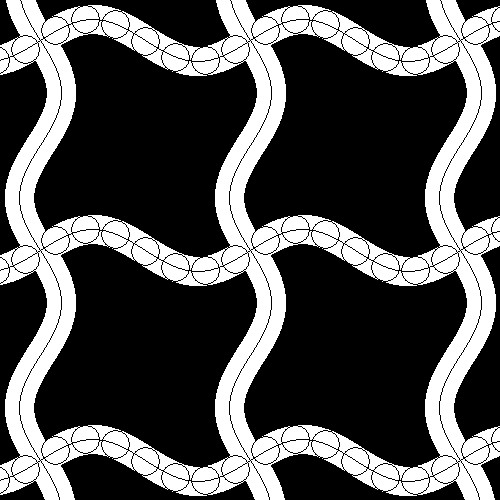Fig 1. Painting the 'sinus' grid by a virtual pen. The mark of the pen is a circular disk (symbolized by the circles) with D diameter. D is equal to the width of the 'streets' (or lines) of the grid. The trace of the center of the virtual pen is a sine curve; this is the middle line of the street. The distortion level is defined as the amplitude of the sine curve ( i.e. the maximal + or - swing from the straight). It can be seen that the  amplitude of the (upper, lower or left, right) edges of the streets are equal to the amplitude of the middle line. Therefore an equivalent definition: the distortion level is the amplitude of  the (upper, lower or  right, left) edges of the streets. N.B.1: The form of the edges is not sine wave; only the middle line is sine wave. N.B.2: If the amplitude of a sine wave is 1, then it swings between -1 to +1.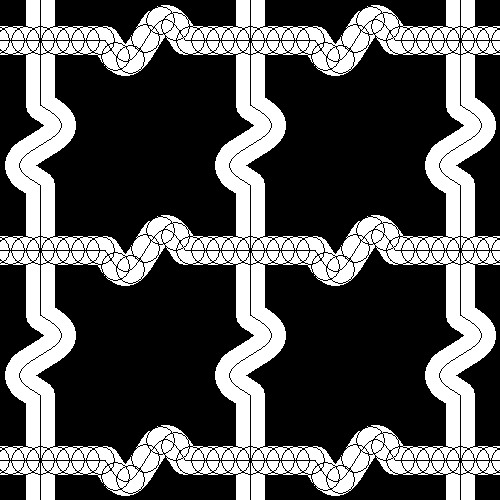Fig 2. Painting the 'wave' grid by a virtual pen. The mark of the pen is a circular disk (symbolized by the circles) with D diameter. D is equal to the width of the 'streets' of the grid. The trace of the center of the virtual pen (i.e. the middle line of the street) consists of  two straight lines and a sine curve with one period. The distortion level is defined as the amplitude of the sine curve (i.e. the maximal + or - swing from the straight). It can be seen that the  amplitude of  the (upper, lower or left, right) edges of the streets are equal to the amplitude of the middle line. Therefore an equivalent definition: the distortion level is the amplitude of  the (upper, lower or  right, left) edges of the streets.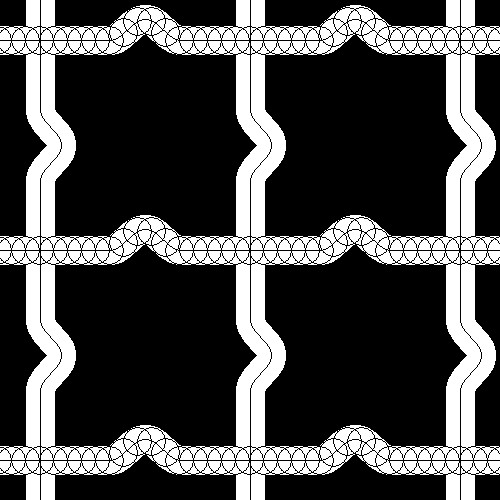Fig 3. Painting the 'hump' grid by a virtual pen. The mark of the pen is a circular disk (symbolized by the circles) with D diameter. D is equal to the width of the 'streets' of the grid. The trace of the center of the virtual pen (i.e.the middle line of the street ) consists of two straight lines and a 'hump'. The distortion level is defined as the amplitude of the middle line;  i.e. the maximal + swing from the straight). It can be seen that the amplitude of  the (upper or  right) edges of the streets are equal to the amplitude of the middle line. Therefore an equivalent definition: the distortion level is the amplitude of  the upper or right edges of the streets.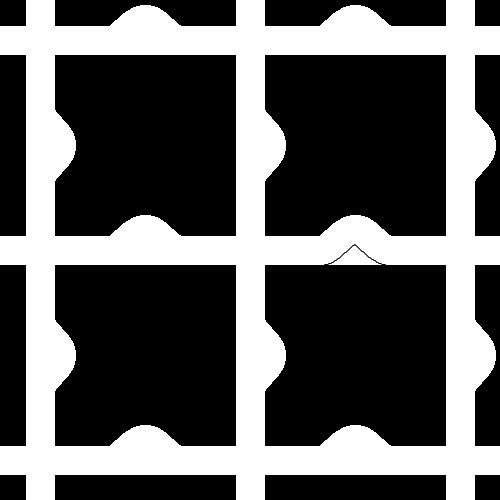Fig 4. The "half hump' grid. It was generated from the 'hump' grid (see Fig 3) by replacing the lower (or left) curve by a straight edge segment. The distortion level is defined as the distortion level of the original 'hump' grid.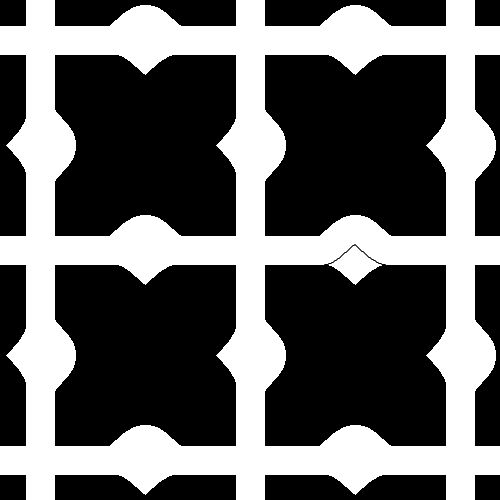Fig 5. The 'asymmetrical hump' grid. It was generated from the 'hump' grid (see fig 3), by  mirroring its lower (or left) curve along the edge of the street. The distortion level is defined as the distortion level of the original 'hump' grid.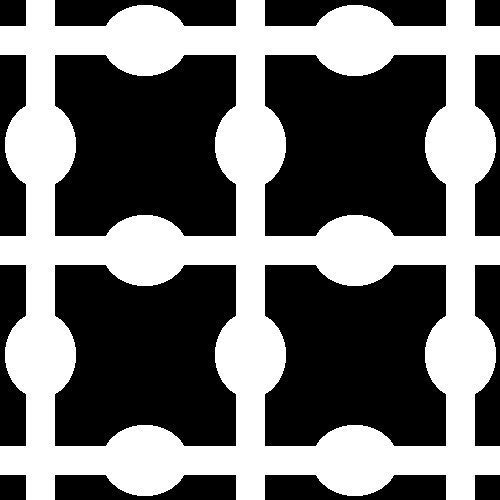Fig 6. Painting the 'knot' grid. There are painted elliptical 'balls'  in the middle part of the street segments  The distortion level is defined as the amplitude of  the (upper, lower or  right, left) edges of the streets.since 2008-06-09